Q1   What is a particle?

A particle has the attributes of mass, momentum, and thus kinetic energy. A particle may carry an electric charge. Particles of atomic size or larger have a definite location in space, and thus are discrete and countable. As particle masses fall below atomic levels, their positions in space and their velocities become  increasingly indeterminate in the sense that the more precisely one (such as the velocity) can be specified, the loss precisely can the other (such as the momentum, and thus its kinetic energy) can be  known, owing to quantum indeterminancy (see Q14 below.) It is these tiny "quantum particles" such as protons (hydrogen nuclei) and especially electrons, that we are especially concerned with in this unit.

Q2   What is a wave?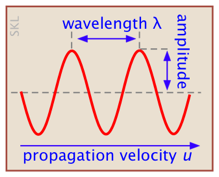A wave is a periodic variation of some quantity as a function of time or location. For example, the wave motion of a vibrating guitar string is defined by the displacement of the string from its center as a function of distance along the string. A sound wave consists of variations in the pressure with location. A wave is characterized by its amplitude, wavelength λ (lambda) and frequency ν (nu). The latter two quantities are connected by the velocity u at which the wave passes through a given medium: u = νλ.

The square of the amplitude gives the intensity of the wave: the energy it conveys per unit time.

Using a sound wave as a simple example, consider the musical note A just below middle C on the piano keyboard, which is commonly tuned to a frequency ν = 440 hz. Given that the velocity of sound in the air is 330 m s–1,
the wavelength of A-440 is λ = (330 m —1) ÷ (440—1 s) = 0.75 m

Q3   What is light?

Phrasing the question in this way reflects the deterministic mode of Western thought which assumes that something cannot "be" two quite different things at the same time. The short response to this question is that all we know about light (or anything else, for that matter) are the results of experiments, and that some kinds of experiments show that light behaves like particles, and that other experiments reveal light to have the properties of waves. For the moment, it is better to amend this question to that given in the next section:

Q4   What is the wave theory light?

In the early 19th century, the English scientist Thomas Young carried out the famous two-slit experiment which demonstrated that a beam of light, when split into two beams and then recombined, will show interference effects that can only be explained by assuming that light is a wavelike disturbance. By 1820, Augustin Fresnel had put this theory on a sound mathematical basis, but the exact nature of the waves remained unclear until the 1860's when James Clerk Maxwell developed his electromagnetic theory.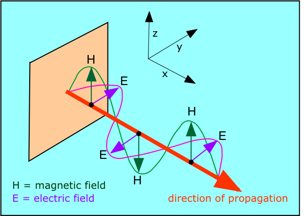From the laws of electromagnetic induction that were discovered in the period 1820-1830 by Hans Christian Oersted and Michael Faraday, it was known that a moving electric charge gives rise to a magnetic field, and that a changing magnetic field can induce electric charges to move. Maxwell showed theoretically that when an electric charge is accelerated (by being made to oscillate within a piece of wire, for example), electrical energy will be lost, and an equivalent amount of energy is radiated into space, spreading out as a series of waves extending in all directions.

What is actually "waving" in electromagnetic radiation? According to Maxwell, it is the strengths of the electric and magnetic fields as they travel through space. The two fields are oriented at right angles to each other and to the direction of travel.

As the electric field changes, it induces a magnetic field, which then induces a new electric field, etc., allowing the wave to propagate itself through space.

These waves consist of periodic variations in the electrostatic and electromagnetic field strengths. These variations occur at right angles to each other. Each electrostatic component of the wave induces a magnetic component, which then creates a new electrostatic component, so that the wave, once formed, continues to propagate through space, essentially feeding on itself. In one of the most brilliant mathematical developments in the history of science, Maxwell expounded a detailed theory, and even showed that these waves should travel at about 3E8 m s–1, a value which experimental observations had shown corresponded to the speed of light. In 1887, the German physicist Heinrich Hertz demonstrated that an oscillating electric charge (in what was in essence the world's first radio transmitting antenna) actually does produce electromagnetic radiation just as Maxwell had predicted, and that these waves behave exactly like light.

It is now understood that light is electromagnetic radiation that falls within a range of wavelengths that can be perceived by the eye. The entire electromagnetic spectrum runs from radio waves at the long-wavelength end, through heat, light, X-rays, and to gamma radiation.

Q5   What events led to the quantum theory of light?

It did not arise from any attempt to explain the behavior of light itself; by 1890 it was generally accepted that the electromagnetic theory could explain all of the properties of light that were then known.

But at just about that time, experiments were conducted that showed that when a metallic electrode is illuminated with light of sufficiently short wavelength, electrons could sometimes be emitted from it; moreover, the kinetic energy of the emitted electrons depends not on the intensity of the light, but on its wavelength, increasing as the wavelength becomes shorter. This phenomenon became known as the photoelectric effect. The German physicist Max Planck who was at the time studying temperature-induced "black body" electromagnetic radiation, suggested, on theoretical grounds, that the energies of these waves did not vary continuously, but rather in discrete steps that we now know as quanta.

Finally, in 1905, Albert Einstein, interpreting these and other experimental results, published his famous paper showing that light can be regarded as a stream of "packets" (i.e., particles, each possessing a discrete kinetic energy.

These events are described in greater detail in Q6-Q9.

Q6   What is black body radiation?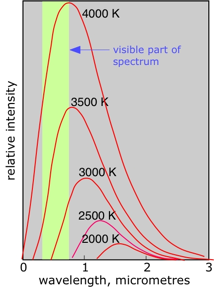All objects above the temperature of absolute zero emit electromagnetic radiation consisting of a broad range of wavelengths described by a distribution curve whose peak wavelength λ (lambda) at absolute temperature T for a "perfect radiator" known as a black body is given by Wein's law which predicts that λmax is inversely proportional to T.

At ordinary temperatures this radiation is entirely in the infrared region of the spectrum, but as the temperature rises above about 1000K, more energy is emitted in the visible wavelength region and the object begins to glow, first with red light, and then shifting toward the blue as the temperature is increased.

This type of radiation has two important characteristics. First, the spectrum is a continuous one, meaning that all wavelengths are emitted, although with intensities that vary smoothly with wavelength. The other curious property of black body radiation is that it is independent of the composition of the object; all that is important is the temperature.

Black body radiation, like all electromagnetic radiation, must originate from oscillations of electric charges which in this case were assumed to be the electrons within the atoms of an object acting somewhat as miniature Hertzian oscillators. It was presumed that since all wavelengths seemed to be present in the continuous spectrum of a glowing body, these tiny oscillators could send or receive any portion of their total energy. However, all attempts to predict the actual shape of the emission spectrum of a glowing object on the basis of classical physical theory proved futile.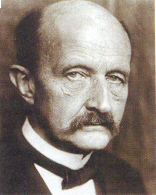In 1900, the great German physicist Max Planck (who earlier in the same year had worked out an empirical formula giving the detailed shape of the black body emission spectrum) showed that the shape of the observed spectrum could be exactly predicted if the energies emitted or absorbed by each oscillator were restricted to integer values of hν, where ν ("nu") is the frequency and h is a constant 6.626E–34 J s which we now know as Planck's Constant. This means that the allowable energies of each oscillator are quantized. (Owing to the minute differences in frequency among the uncountable numbers of oscillators in any visible body, the spectrum appears to be continuous.) This modification of classical theory, the first use of the quantum concept, was as unprecedented as it was simple, and it set the stage for the development of modern quantum physics.

Q8   What is the photoelectric effect?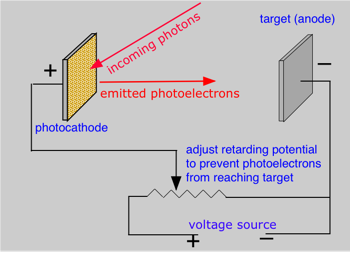Shortly after J.J. Thompson's experiments led to the identification of the elementary charged particles we now know as electrons, it was discovered that the illumination of a metallic surface by light can cause electrons to be emitted from the surface. This phenomenon, the photoelectric effect, is studied by illuminating one of two metal plates in an evacuated tube. The kinetic energy of the photoelectrons causes them to move to the opposite electrode, thus completing the circuit and producing a measurable current. However, if an opposing potential (the retarding potential) is imposed between the two plates, the kinetic energy can be reduced to zero so that the electron current is stopped. By observing the value of the retarding potential Vr, the kinetic energy of the photoelectrons can be calculated from the electron charge e, its mass m and the frequency ν of the incident light: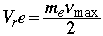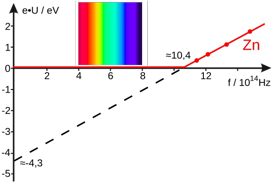This plot illustrates the photoelectric effect in metallic zinc. No photoelectrons are emitted until the frequency of the incident light reaches 10.4 ×1014 hz = 1.04 × 1015 hz. This frequency corresponds to a wavelength of

(3E8 m s—1) / (1.04E15 s—1) =288E-6 m

which, as the diagram indicates, falls outside the range of visible light, in the ultraviolet region of the spectrum. [image from Wikimedia Commons]

Q9   What peculiarity of the photoelectric effect led to the idea of the photon?

Although the number of electrons ejected from the metal surface per second depends on the intensity of the light, as expected, the kinetic energies of these electrons (as determined by measuring the retarding potential needed to stop them) does not, and this was definitely not expected. Just as a more intense physical disturbance will produce higher energy waves on the surface of the ocean, it was supposed that a more intense light beam would confer greater energy on the photoelectrons. But what was found, to everyone's surprise, is that the photoelectron energy is controlled by the wavelength of the light, and that there is a critical wavelength below which no photoelectrons are emitted at all.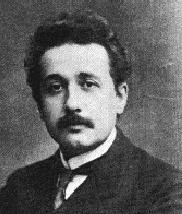Albert Einstein quickly saw that if the kinetic energy of the photoelectrons depends on the wavelength of the light, then so must its energy. Further, if Planck was correct in supposing that energy must be exchanged in packets restricted to certain values, then light must similarly be organized into energy packets. But a light ray consists of electric and magnetic fields that spread out in a uniform, continuous manner; how can a continuously-varying wave front exchange energy in discrete amounts? Einstein's answer was that the energy contained in each packet of the light must be concentrated into a tiny region of the wave front. This is tantamount to saying that light has the nature of a quantized particle whose energy is given by the product of Planck's constant and the frequency: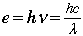Einstein's publication of this explanation in 1905 led to the rapid acceptance of Planck's idea of energy quantization, which had not previously attracted much support from the physics community of the time. It is interesting to note, however, that this did not make Planck happy at all. Planck, ever the conservative, had been reluctant to accept that his own quantized-energy hypothesis was much more than an artifice to explain black-body radiation; to extend it to light seemed an absurdity that would negate the well-established electromagnetic theory and would set science back to the time before Maxwell.

Q10   What role does relativity play in atoms?

Einstein's special theory of relativity arose from his attempt to understand why the laws of physics that describe the current induced in a fixed conductor when a magnet moves past it are not formulated in the same way as the ones that describe the magnetic field produced by a moving conductor. The details of this development are not relevant to our immediate purpose, but some of the conclusions that this line of thinking led to very definitely are. Einstein showed that the velocity of light, unlike that of a material body, has the same value no matter what velocity the observer has. Further, the mass of any material object, which had previously been regarded as an absolute, is itself a function of the velocity of the body relative to that of the observer (hence "relativity"), the relation being given by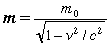in which mo is the rest mass of the particle, v is its velocity with respect to the observer, and c is the velocity of light.

According to this formula, the mass of an object increases without limit as the velocity approaches that of light. Where does the increased mass come from? Einstein's answer was that the increased mass is that of the kinetic energy of the object; that is, energy itself has mass, so that mass and energy are equivalent according to the famous formula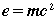The only particle that can move at the velocity of light is the photon itself, due to its zero rest mass.

Q11   If the photon has no mass, how can it have a momentum?

Although the photon has no rest mass, it does possess kinetic energy by virtue ot its velocity. The mass-equivalent of this energy is given by Einstein's relation m = e/c2. Combining this with Planck's relation e = hν, the effctive mass of the photon becomes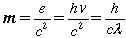which corresponds to a momentum m v of (h/cλ) × c = h/λ .

Q12   If waves can be particles, can particles be waves?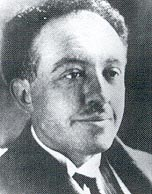In 1924, the French physicist Louis deBroglie proposed (in his doctoral thesis) that just as light possesses particle-like properties, so should particles of matter exhibit a wave-like character. Within two years this hypothesis had been confirmed experimentally by observing the diffraction (a wave interference effect) produced by a beam of electrons as they were scattered by the row of atoms at the surface of a metal.

deBroglie showed that the wavelength of a particle is inversely proportional to its momentum: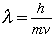Notice that the wavelength of a stationary particle is infinitely large, while that of a particle of large mass approaches zero. For most practical purposes, the only particle of interest to chemistry that is sufficiently small to exhibit wavelike behaveior is the electron (mass 9.11E­31 kg).

Q13   Exactly what aspect of a particle can be said to be "waving"?

In 1927, Werner Heisenberg proposed that certain pairs of properties of a particle cannot simultaneously have exact values. In particular, the position and the momentum of a particle have associated with them uncertaintiesx andp given by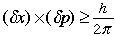As with the de Broglie particle wavelength, this has practical consequences only for electrons and other particles of very small mass. It is very important to understand that these "uncertainties" are not merely limitations related to experimental error or observational technique, but instead they express an underlying fact that Nature does not allow a particle to possess definite values of position and momentum at the same time. This principle (which would be better described by the term "indeterminacy" than "uncertainty") has been thoroughly verified and has far-reaching practical consequences which extend to chemical bonding and molecular structure. For an interactive demonstration, see here.

Q14   What is the uncertainty principle?

In 1927, Werner Heisenberg proposed that certain pairs of properties of a particle cannot simultaneously have exact values. In particular, the position and the momentum of a particle have associated with them uncertaintiesx andp given byAs with the de Broglie particle wavelength, this has practical consequences only for electrons and other particles of very small mass. It is very important to understand that these "uncertainties" are not merely limitations related to experimental error or observational technique, but instead they express an underlying fact that Nature does not allow a particle to possess definite values of position and momentum at the same time. This principle (which would be better described by the term "indeterminacy" than "uncertainty") has been thoroughly verified and has far-reaching practical consequences which extend to chemical bonding and molecular structure. For an interactive demonstration, see here.

Q15   Is the uncertainty principle consistent with particle waves?

Yes; either one really implies the other. Consider the following two limiting cases: · A particle whose velocity is known to within a very small uncertainty will have a sharply-defined energy (because its kinetic energy is known) which can be represented by a probability wave having a single, sharply-defined frequency. A"monochromatic" wave of this kind must extend infinitely in space: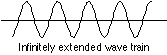But if the peaks of the wave represent locations at which the particle is most likely to manifest itself, we are forced to the conclusion that it can "be" virtually anywhere, since the number of such peaks is infinite! Now think of the opposite extreme: a particle whose location is closely known. Such a particle would be described by a short wavetrain having only a single peak, the smaller the uncertainty in position, the more narrow the peak.

To help you see how waveforms of different wavelength combine, two such combinations are shown below: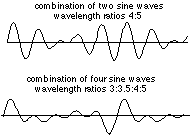It is apparent that as more waves of different frequency are mixed, the regions in which they add constructively diminish in extent. The extreme case would be a wavetrain in which destructive interference occurs at all locations except one, resulting in a single pulse: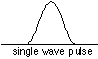Is such a wave possible, and if so, what is its wavelength? Such a wave is possible, but only as the sum (interference) of other waves whose wavelengths are all slightly different. Each component wave possesses its own energy (momentum), and adds that value to the range of momenta carried by the particle, thus increasing the uncertainty δp. In the extreme case of a quantum particle whose location is known exactly, the probability wavelet would have zero width which could be achieved only by combining waves of all wavelengths-- an infinite number of wavelengths, and thus an infinite range of momentum δp and thus kinetic energy.

Q16   So should we call them particles or waves?

The short answer is that the experiments described below reveal that matter having a very small mass (usually referred to as "quantum particles") can exhibit the properties of particles or waves, depending on the experiment; so the "either-or" dichotomy that applies to the macro world becomes meaningless.

Suppose we direct a beam of photons (or electrons; the experiment works with both) toward a piece of metal having a narrow opening. On the other side there are two more openings, or slits. Finally the particles impinge on a photographic plate or some other recording device. Taking into account their wavelike character, we would expect the probability waves to produce an interference pattern of the kind that is well known for sound and light waves, and this is exactly what is observed; the plate records a series of alternating dark and light bands, thus demonstrating beyond doubt that electrons and light have the character of waves.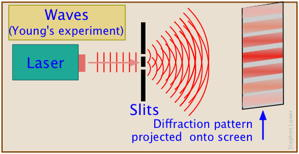Now let us reduce the intensity of the light so that only one photon at a time passes through the apparatus (it is experimentally possible to count single photons, so this is a practical experiment). Each photon passes through the first slit, and then through one or the other of the second set of slits, eventually striking the photographic film where it creates a tiny dot. If we develop the film after a sufficient number of photons have passed through, we find the very same interference pattern we obtained previously.

There is something strange here. Each photon, acting as a particle, must pass through one or the other of the pair of slits, so we would expect to get only two groups of spots on the film, each opposite one of the two slits. Instead, it appears that the each particle, on passing through one slit, "knows" about the other, and adjusts its final trajectory so as to build up a wavelike interference pattern.

It gets even stranger: suppose that we set up a detector to determine which slit a photon is heading for, and then block off the other slit with a shutter. We find that the photon sails straight through the open slit and onto the film without trying to create any kind of an interference pattern. Apparently, any attempt to observe the photon as a discrete particle causes it to behave like one.

The only conclusion possible is that quantum particles have no well defined paths; each photon (or electron) seems to have an infinity of paths which thread their way through space, seeking out and collecting information about all possible routes, and then adjusting its behavior so that its final trajectory, when combined with that of others, produces the same overall effect that we would see from a train of waves of wavelength λ= h/mv.

Q17   What are line spectra?

We have already seen that a glowing body (or actually, any body whose temperature is above absolute zero) emits and absorbs radiation of all wavelength in a continuous spectrum. In striking contrast is the spectrum of light produced when certain substances are volatilized in a flame, or when an electric discharge is passed through a tube containing gaseous atoms of an element. The light emitted by such sources consists entirely of discrete wavelengths. This kind of emission is known as a discrete spectrum or line spectrum (the "lines" that appear on photographic images of the spectrum are really images of the slit through which the light passes before being dispersed by the prism in the spectrograph).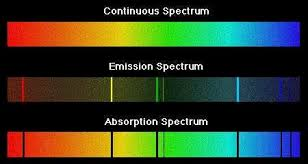Contrast the two line spectra (bottom) in this image with the continuous spectrum at the top. As its name implies, a continuous spectrum contains all wavelengths, as in the light emitted by a hot body such as a tungsten lamp filament, or the Sun.

Emission line spectra are produced by atoms in which electronic excitation (input of energy produced by electric discharges or extreme heat) has temporarily knocked electrons out of their stable shells into higher, unstable orbitals.  After a few nanosecons, these electrons fall back into their stable states, emitting light whose wavelength corresponds to the [quantized] energy differences beween the excited and "ground" (normal) states.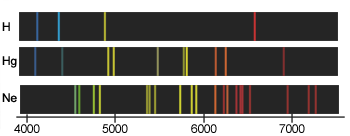Every element has its own line spectrum which serves as a sensitive and useful tool for detecting the presence and relative abundance of the element, not only in terrestrial samples but also in stars. (As a matter of fact, the element helium was discovered in the sun, through its line spectrum, before it had been found on Earth.) In some elements, most of the energy in the visible part of the emission spectrum is concentrated into just a few lines, giving their light characteristic colors: yellow-orange for sodium, blue-green for mercury (these are commonly seen in street lights) and orange for neon.

Line spectra were well known early in the 19th century, and were widely used for the analysis of ores and metals. The German spectroscopist R.W. Bunsen, now famous for his gas burner, was then best known for discovering two new elements, rubidium and cesium, from the line spectrum he obtained from samples of mineral spring waters.

Q18   How are line spectra organized?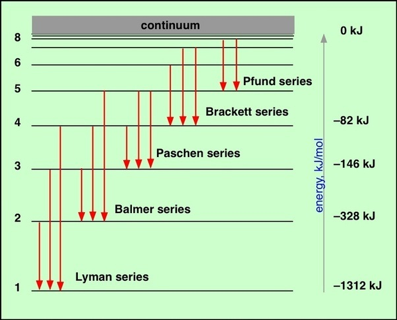Until 1885, line spectra were little more than "fingerprints" of the elements; extremely useful in themselves, but incapable of revealing any more than the identify of the individual atoms from which they arise. In that year a Swiss school teacher named Johann Balmer published a formula that related the wavelengths of the four known lines in the emission spectrum of hydrogen in a simple way. Balmer's formula was not based on theory; it was probably a case of cut-and-try, but it worked: he was able to predict the wavelength of a fifth, yet-to-be discovered emission line of hydrogen, and as spectroscopic and astronomical techniques improved (the only way of observing highly excited hydrogen atoms at the time was to observe the solar spectrum during an eclipse), a total of 35 lines were discovered, all having wavelengths given by the formula which we write in the modern manner as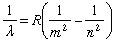in which m = 2 and R is a constant (the Rydberg constant, after the Swedish spectroscopist) whose value is 1.09678E7 m–1. The variable n is an integer whose values 1, 2, etc. give the wavelengths of the different lines.

It was soon discovered that by replacing m with integers other than 2, other series of hydrogen lines could be accounted for. These series, which span the wavelength region from the ultraviolet through infrared, are named after their discoverers.

Attempts to adapt Balmer's formula to describe the spectra of atoms other than hydrogen generally failed, although certain lines of some of the spectra seemed to fit this same scheme, with the same value of R.

Q19   How large can n be?

There is no limit; values in the hundreds have been observed, although doing so is very difficult because of the increasingly close spacing of successive levels as n becomes large. Atoms excited to very high values of n are said to be in Rydberg states.

Q20   Why do line spectra become continuous at short wavelengths?

As n becomes larger, the spacing between neighboring levels diminishes and the discrete lines merge into a continuum. This can mean only one thing: the energy levels converge as n approaches infinity. This convergence limit corresponds to the energy required to completely remove the electron from the atom; it is the ionization energy.At energies in excess of this, the electron is no longer bound to the rest of the atom, which is now of course a positive ion. But an unbound system is not quantized; the kinetic energy of the ion and electron can now have any value in excess of the ionization energy. When such an ion and electron pair recombine to form a new atom, the light emitted will have a wavelength that falls in the continuum region of the spectrum. Spectroscopic observation of the convergence limit is an important method of measuring the ionization energies of atoms.

Q21   What were the problems with the planetary model of the atom?

Rutherford's demonstration that the mass and the positive charge of the atom is mostly concentrated in a very tiny region called the nucleus forced the question of just how the electrons are disposed outside the nucleus. By analogy with the solar system, a planetary model was suggested: if the electrons were orbiting the nucleus, there would be a centrifugal force that could oppose the electrostatic attraction and thus keep the electrons from falling into the nucleus. This of course is similar to the way in which the centrifugal force produced by an orbiting planet exactly balances the force due to its gravitational attraction to the sun.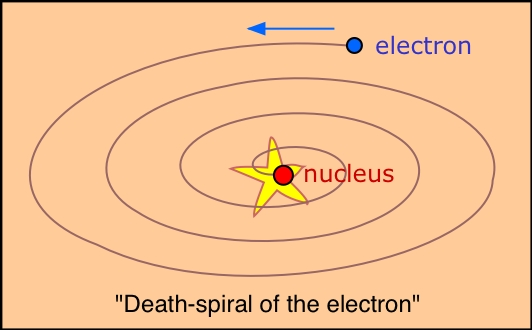The planetary model suffers from one fatal weakness: electrons, unlike planets, are electrically charged. An electric charge revolving in an orbit is continually undergoing a change of direction, that is, acceleration. It has been well known since the time of Hertz that an accelerating electric charge radiates energy. We would therefore expect all atoms to act as miniature radio stations. Even worse, conservation of energy requires that any energy that is radiated must be at the expense of the kinetic energy of the orbital motion of the electron. Thus the electron would slow down, reducing the centrifugal force and allowing the electron to spiral closer and closer to the nucleus, eventually falling into it. In short, no atom that operates according to the planetary model would last long enough for us to talk about it.

As if this were not enough, the planetary model was totally unable to explain any of the observed properties of atoms, including their line spectra.

Q22   How did Bohr's theory save the planetary model... for a while?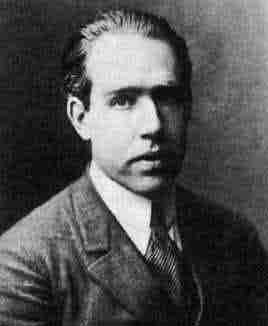Niels Bohr was born in the same year (1885) that Balmer published his formula for the line spectrum of hydrogen. Beginning in 1913, the brilliant Danish physicist published a series of papers that would ultimately derive Balmer's formula from first principles.

Bohr's first task was to explain why the orbiting electron does not radiate energy as it moves around the nucleus. This energy loss, if it were to occur at all, would do so gradually and smoothly. But Planck had shown that blackbody radiation could only be explained if energy changes were limited to jumps instead of gradual changes. If this were a universal characteristic of energy- that is, if all energy changes were quantized, then very small changes in energy would be impossible, so that the electron would in effect be "locked in" to its orbit.

From this, Bohr went on to propose that there are certain stable orbits in which the electron can exist without radiating and thus without falling into a "death spiral". This supposition was a daring one at the time because it was inconsistent with classical physics, and the theory which would eventually lend it support would not come along until the work of de Broglie and Heisenberg more than ten years later.

Since Planck's quanta came in multiples of h, Bohr restricted his allowed orbits to those in which the product of the radius r and the momentum of the electron mv (which has the same units as h: J–s) are integral multiples of h:

2πrmv = nh     (n = 1,2,3, . .)

Each orbit corresponds to a different energy, with the electron normally occupying the one having the lowest energy, which would be the innermost orbit of the hydrogen atom.

Taking the lead from Einstein's explanation of the photoelectric effect, Bohr assumed that each spectral line emitted by an atom that has been excited by absorption of energy from an electrical discharge or a flame represents a change in energy given by ΔE = hn hc/λ, the energy lost when the electron falls from a higher orbit (value of n) into a lower one.

Finally, as a crowning triumph, Bohr derived an expression giving the radius of the nth orbit for the electron in hydrogen as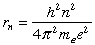Substitution of the observed values of the electron mass and electron charge into this equation yielded a value of 0.529E­10 m for the radius of the first orbit, a value that corresponds to the radius of the hydrogen atom obtained experimentally from the kinetic theory of gases. Bohr was also able to derive a formula giving the value of the Rydberg constant, and thus in effect predict the entire emission spectrum of the hydrogen atom.

Q23   What were the main problems with Bohr's theory?

There were two kinds of difficulties. First, there was the practical limitation that it only works for atoms that have one electron-- that is, for H, He+, Li2+, etc. The second problem was that Bohr was unable to provide any theoretical justification for his assumption that electrons in orbits described by the preceding equation would not lose energy by radiation. This reflects the fundamental underlying difficulty: because de Broglie's picture of matter waves would not come until a decade later, Bohr had to regard the electron as a classical particle traversing a definite orbital path.

Bohr's theory and its elaborations

Q24   How did the wave picture of the electron save Bohr's theory?

Once it became apparent that the electron must have a wavelike character, things began to fall into place. The possible states of an electron confined to a fixed space are in many ways analogous to the allowed states of a vibrating guitar string. These states are described as standing waves that must possess integral numbers of nodes. The states of vibration of the string are described by a series of integral numbers n = 1,2,... which we call the fundamental, first overtone, second overtone, etc. The energy of vibration is proportional to n2. Each mode of vibration contains one more complete wave than the one below it.

In exactly the same way, the mathematical function that defines the probability of finding the electron at any given location within a confined space possesses n peaks and corresponds to states in which the energy is proportional to n2.

The electron in a hydrogen atom is bound to the nucleus by its spherically symmetrical electrostatic charge, and should therefore exhibit a similar kind of wave behavior. This is most easily visualized in a two-dimensional cross section that corresponds to the conventional electron orbit. But if the particle picture is replaced by de Broglie's probability wave, this wave must follow a circular path, and- most important of all- its wavelength (and consequently its energy) is restricted to integral multiples n = 1,2,.. of the circumference 2πr = nλ.

for otherwise the wave would collapse owing to self-interference. That is, the energy of the electron must be quantized; what Bohr had taken as a daring but arbitrary assumption was now seen as a fundamental requirement. Indeed the above equation can be derived very simply by combining Bohr's quantum condition 2πrmv = nh with the expression mv = h/λ for the deBroglie wavelength of a particle.

Q25   What is an orbital?

Because the classical view of an electron as a localizable particle is now seen to be untenable, so is the concept of a definite trajectory, or "orbit". Instead, we now use the word orbital to describe the state of existence of an electron. An orbital is really no more than a mathematical function describing the standing wave that gives the probability of the electron manifesting itself at any given location in space. More commonly (and loosely) we use the word to describe the region of space in which an electron is likely to be found. Each kind of orbital is characterized by a set of quantum numbers n, l, and m These relate, respectively, to the average distance of the electron from the nucleus, to the shape of the orbital, and to its orientation in space.

Q26   If the electron cannot be localized, can it be moving?

In its lowest state in the hydrogen atom (in which l=0) the electron has zero angular momentum, so electrons in s orbitals are not in motion. In orbitals for which l>0 the electron does have an effective angular momentum, and since the electron also has a definite rest mass me = 9.11E­31 kg, it must possess an effective velocity. Its value can be estimated from the Uncertainty Principle; if the volume in which the electron is confined is about 10–10 m, then the uncertainty in its momentum is at least h/(1010) = 6.6E–24 kg m s–1, which implies a velocity of around 107 m s–1, or almost one-tenth the velocity of light.

The stronger the electrostatic force of attraction by the nucleus, the faster the effective electron velocity. In fact, the innermost electrons of the heavier elements have effective velocities so high that relativistic effects set in; that is, the effective mass of the electron significantly exceeds its rest mass. This has direct chemical effects; it is the cause, for example, of the low melting point of metallic mercury and of the color of gold.

Q27   Why does the electron not fall into the nucleus?

The following is a very brief answer; for a more complete explanation, please see
"How the battle of the infinities saves the electron from its death spiral".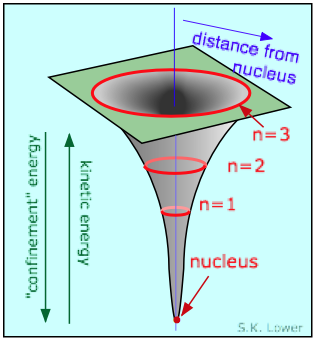The negatively-charged electron is attracted to the positive charge of the nucleus. What prevents it from falling in? This question can be answered in various ways at various levels. All start with the statement that the electron, being a quantum particle, has a dual character and cannot be treated solely by the laws of Newtonian mechanics.

We saw above that in its wavelike guise, the electron exists as a standing wave which must circle the nucleus at a sufficient distance to allow at least one wavelength to fit on its circumference. This means that the smaller the radius of the circle, the shorter must be the wavelength of the electron, and thus the higher the energy. Thus it ends up "costing" the electron energy if it gets too close to the nucleus. The normal orbital radius represents the balance between the electrostatic force trying to pull the electron in, and what we might call the "confinement energy" that opposes the electrostatic energy. This confinement energy can be related to both the particle and wave character of the electron.

If the electron as a particle were to approach the nucleus, the uncertainty in its position would become so small (owing to the very small volume of space close to the nucleus) that the momentum, and therefore the energy, would have to become very large. The electron would, in effect, be "kicked out" of the nuclear region by the "confinement" energy.

Q28   What is electron spin?

Each electron in an atom has associated with it a magnetic field whose direction is quantized; there are only two possible values that point in opposite directions. We usually refer to these as "up" and "down", but the actual directions are parallel and antiparallel to the local magnetic field associated with the orbital motion of the electron.

The term spin is somewhat misleading; it implies that this magnetic moment is produced by the electron charge as the electron rotates about its own axis. Although this conveys a vivid mental picture of the source of the magnetism, the electron is not an extended body and its "rotation" is meaningless. Electron spin has no classical counterpart and no simple explanation; the magnetic moment is a consequence of relativistic shifts in local space and time due to the high effective velocity of the electron in the atom. This effect was predicted theoretically by P.A.M. Dirac in 1928.